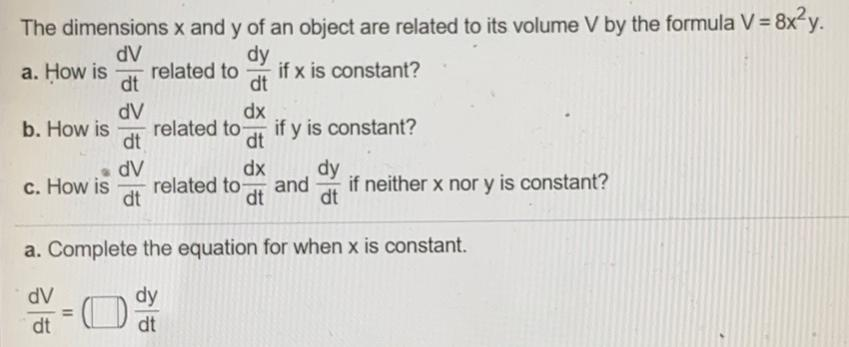Question:

# The dimensions x and y of an object are related to its

Last updated: 7/27/2022The dimensions x and y of an object are related to its volume V by the formula V = 8x²y. a. How is dV/dt related to dy/dt if x is constant? b. How is dV/dt related to dx/dt if y is constant? c. How is dV/dt related to dx/dt and dy/dt if neither x nor y is constant?